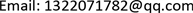1. 引言

2. 开关电路拓扑结构及其原理分析

CCM模式下，电路主要器件的电流波形如图3所示。具体模态分析如下所述。

3. 稳态特性分析3.1. 变换器电压增益

v L m = V i n (1)

v N 2 = n V i n (2)

v L m = − V C 1 (3)

v N 2 = − V C 2 + V C 1 (4)

∫ 0 D T S V i n d t + ∫ D T S T S ( − V C 1 ) d t = 0 (5)

∫ 0 D T S ( n V i n ) d t + ∫ D T S T S ( − V C 2 + V C 1 ) d t = 0 (6)

V C 1 = D 1 − D V i n (7)

V C 2 = ( n + 1 ) D 1 − D V i n (8)

V o = n + 1 1 − D V i n (9)

3.2. 功率器件的电压应力

V d s = V i n + V C 1 = 1 n + 1 V O (10)

V D 1 = V i n + V C 1 = 1 n + 1 V O (11)

V D 2 = V C 2 + V N 2 − V C 1 = n n + 1 V O (12)

V D 3 = V C 3 = V o (13)

4. 仿真验证

Parameters of circui

5. 总结